Visitors Online: 77 | Saturday 25th May 2019

CBSE Guess > Papers > Question Papers > Class XII > 2004 > Chemistry > Outside Delhi Set-I

CHEMISTRY (Set I—Outside Delhi)

Q. 1. Define the term 'amorphous'. 1

Q. 2. What is the sum of the mole fractions of all the components in a three compo-nent system? 1

Q. 3. What is meant by an elementary reaction? 1

Q. 4. Write the IUPAC name the following: 1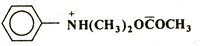Q. 5. Draw the structure of 4-methylpent-3-en-2-one. 1

Q. 6. Compare the relative stability of the following species and indicate their mag-netic (diamagnetic or paramagnetic) properties: 2Q. 7. How does molar conductivity vary with concentration for (i) weak electrolyte and for (ii) strong electrolyte? Give reasons for these variations. 2

Q. 8. Give the chemical reactions as an evidence for each of the following observa-tions. 2
(i) Tin ( II ) is a reducing agent wheras Pb ( II ) is not.
(ii) +1 gallium undergoes disproportionation reaction.

Q. 9. Identify and indicate the presence of centre of chirality, if any, in the following molecules. How many stereoisomers are possible for those containing chiral centre? 2
(i) 1, 2-dichloropropane
(ii) 3-bromo-pent-I-ene

Q. 10. What happens when ethanol is heated with concentrated sulphuric acid at 453 K? Explain the mechanism of this reaction. 2

Q. 11. Write the mode of free radical addition polymerization of an alkene. Clearly indicate the role of an initiator in it. 2

Q. 12. Write the major classes in which the carbohydrates are divided depending upon whether these undergo hydrolysis, and if so, on the number of products formed. 2

Or

Explain mutarotation taking D-glucose as an example.

Q. 13. What do you understand by (i) Radial probability density, R2 and (ii) Radial probability function,? Draw the curves which show their variation with r for 2s orbital of hydrogen atom. 3

Q. 14. Calculate the value of Avogadro constant from the following data: 3
Density of NaCl = 2.165 g cm--3
Distance between Na+ and CI - in NaCI = 281 pm
(Molar mass of NaCI = 58.5 g mol-1)

Q. 15. The elements A and B form purely covalent compounds having molecular formulae AB2 and AB4 . When dissolved in 20 g of benzene, I g of AB2 lowers the freezing point by 2.3 K, whereas 1 g of AB4 lowers it by 1.3 K. The molar depression constant for benzene is 5.1 K kg mol-1. Calculate the atomic mass of A and atomic mass of B. 3

Q. 16. Write the Nernst equation and calculate the e.m.f. of the following cell at 298 K: 3
Cu (s) | Cu2+ (0.130 M) | | Ag+ (1.00 X 10 -4 M) | Ag (s)

Given: E0 Cu2+/Cu = 0.34V and E0Ag+/Ag = +0.80 V.

Q. 17. Following reaction takes place in one step,
2NO (g) + O 2 (g)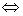2 NO2 (g).
How will the rate of the above reaction change if the volume of the reaction vessel is diminished to one-third of its original volume? Will there be any change in the order of the reaction with the reduced volume? 3

Q. 18. Explain what is observed when 3
(i) an electrolyte is added to ferric hydroxide sol.
(ii) an emulsion is subjected to centrifugation.
(iii) direct current is passed through a colloidal sol.

Or

Define adsorption and write two important differences between physical adsorption and chemisorption.

Q. 19. Draw a figure to show splitting of degenerate d orbitals in an octahedral field. How is the magnitude ofaffected by (i) nature of ligand and (ii) oxidation state of metal ion? 3

Q. 20. Complete the following nuclear reactions: 3
(i)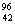Mo(..n)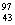Tc

(ii)Cm +C --> ..+4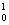n

(iii)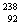U(--> .
(Note Use symbol "X' where element is not known)

Q. 21. Describe simple chemical tests to distinguish between the following pairs of compounds: 3
(i) Propanal ad Propanone
(ii) Phenol and Benzoic acid
(iii) Diethyl ether and Propanol

Q. 22. Give appropriate reasons for each of the following observations: 3
(i) The aromatic amines are weaker bases than aliphatic amines.
(ii) Even under mild conditions aniline on bromination gives 2, 4, 6- tribromoaniline instantaneously.
(iii) The diazonium ion acts as an electrophile.

Q. 23. Enumerate the structural differences between DNA and RNA. Write down the structure of sugar present in DNA. 3

Q. 24. Describe the following with example in each case: 3
(i) Antioxidants
(ii) Biodegradable detergents
(iii) Hybrid propellants

Q. 25. (a) What is meant by an entropy driven reaction? How can a reaction with positive enthalpy and entropy changes be made entropy driven?
(b) Calculate the standard Gibbs energy change for the formation of propane,
C3H8 (g) at 298 K.H0 for propane is -103.85 kJ mol-1.

3C(graphite) +4H2 (g) --> à C3 H8 (g)

Given: S0m C3H8 (g) = 270.2 J K-1 mol-1 ', S0m H2 (g) = 130.68 J K-1 mol-1,

S0m C<(graphite)/sub> = 5.74 J K -1 mol -1.

Q. 26. (i) Give reasons for the following observations:
(a) The tendency for catenation decreases down the group in Group 14.
(b) The decreasing stability of +3 oxidation state with increasing atomic number in Group 13.
(c) SF6 is not easily hydrolysed through thermodynamically it should be.

(ii) Draw the structure of the following species:
(a) (Si2 O7)6-
(b) CIO--4

Or

(i) Assign reasons for the following observations:
(a) PbO2 is a stronger oxidising agent than SnO2.
(b) The bond energy of F2 is less than that of Cl2.
(c) Sulphur in vapour state exhibits paramagnetism.

(ii) Draw the structures of the following species:
(a) (SiO2-3 )n
(b) BrO--4

Q. 27. (a) Describe the variability of oxidation states in the first row of the transition elements (Sc - Cu) and indicate the general trend:
(b) Write chemical reactions Involved In the following:
(a) When matte is charged into silica lined converter in the metallurgy of copper.
(ii) When a solution of chromate is acidified.
(iii) A developed photographic film is subjected of fixing. 5

 Chemistry 2004 Question Papers Class XII Delhi Outside Delhi Compartment Delhi Compartment Outside Delhi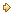Set ISet ISet ISet ISet IISet IISet IISet IISet IIISet III

CBSE 2004 Question Papers Class XII